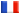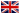# Séminaire de Cryptographie

## Kazuhiro Yokoyama### Usage of Modular Techniques for Efficient Computation of IdealOperation

Modular techniques are widely applied to various algebraic computations. (See  for basic modular techniques applied to polynomial computations.) In this talk, we discuss how modular techniques are efficiently applied to computation of various ideal operations such as Gröbner base computation and ideal decompositions. Here, by *modular techniques* we mean techniques using certain projections for improving the efficiency of the total computation, and by *modular computations*, we mean corresponding computations applied to projected images.

 : von zur Gathen, J., Gerhard, J.: Modern Computer Algebra. Cambridge University Press, Cambridge (1999)